PGF/TikZ Manual

# The TikZ and PGF Packages Manual for version 3.1.10

## Libraries

#### 66 Plot Mark Library

• TikZ Library plotmarks

• \usepgflibrary{plotmarks} % and plain and pure pgf
\usepgflibrary[plotmarks] % Cont and pure pgf
\usetikzlibrary{plotmarks} % and plain when using TikZ
\usetikzlibrary[plotmarks] % Cont when using TikZ

This library defines a number of plot marks.

This library defines the following plot marks in addition to *, x, and + (the filling color has been set to a dark yellow):

.
\pgfuseplotmark{-}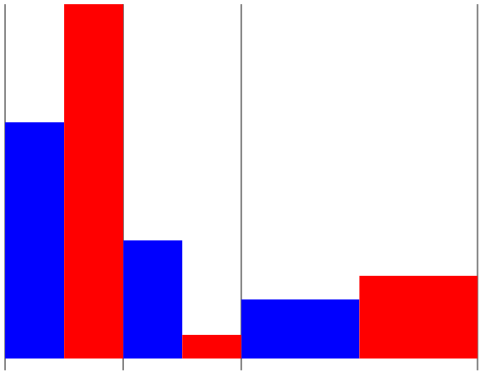\pgfuseplotmark{|}\pgfuseplotmark{o}\pgfuseplotmark{asterisk}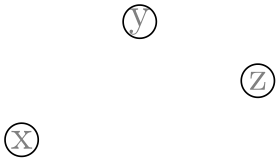\pgfuseplotmark{star}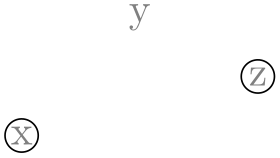\pgfuseplotmark{10-pointed star}\pgfuseplotmark{oplus}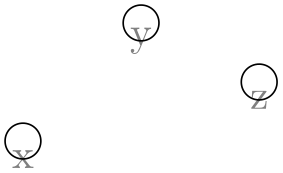\pgfuseplotmark{oplus*}\pgfuseplotmark{otimes}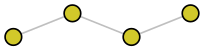\pgfuseplotmark{otimes*}\pgfuseplotmark{square}\pgfuseplotmark{square*}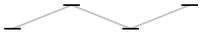\pgfuseplotmark{triangle}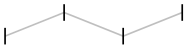\pgfuseplotmark{triangle*}\pgfuseplotmark{diamond}\pgfuseplotmark{diamond*}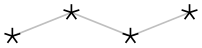\pgfuseplotmark{halfdiamond*}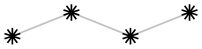\pgfuseplotmark{halfsquare*}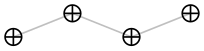\pgfuseplotmark{halfsquare right*}\pgfuseplotmark{halfsquare left*}\pgfuseplotmark{pentagon}\pgfuseplotmark{pentagon*}\pgfuseplotmark{Mercedes star}\pgfuseplotmark{Mercedes star flipped}\pgfuseplotmark{halfcircle}\pgfuseplotmark{halfcircle*}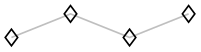\pgfuseplotmark{heart}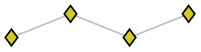\pgfuseplotmark{text}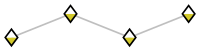Note that each of the provided marks can be rotated freely by means of mark options={rotate=90} or every mark/.append style={rotate=90}.

• /pgf/mark color={color} (no default, initially empty)

• Defines the additional fill color for the halfcircle, halfcircle*, halfdiamond* and halfsquare* markers. An empty value uses white (which is the initial configuration). The special value none disables filling of the respective parts.

Note that halfsquare will be filled with mark color, and the starred variant halfsquare* will be filled half with mark color and half with the actual fill color.

• /pgf/text mark={text} (no default, initially p)

• Changes the text shown by mark=text.

With /pgf/text mark=m: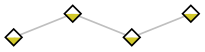With /pgf/text mark=A: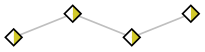There is no limitation about the number of characters or whatever. In fact, any material can be inserted as {text}, including images.

• /pgf/text mark as node={boolean} (no default, initially false)

• Configures how mark=text will be drawn: either as \node or as \pgftext.

The first choice is highly flexible and possibly slow, the second is very fast and usually enough.

• /pgf/text mark style={options for mark=text}(no default)

• Defines a set of options which control the appearance of mark=text.

If /pgf/text mark as node=false (the default), {options} is provided as argument to \pgftext – which provides only some basic keys like left, right, top, bottom, base and rotate.

If /pgf/text mark as node=true, {options} is provided as argument to \node. This means you can provide a very powerful set of options including anchor, scale, fill, draw, rounded corners etc.